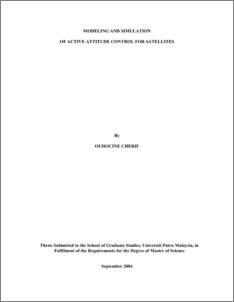# Modeling and Simulation of Active Attitude Control for Satellites

## Citation

Ouhocine, Cherif (2004) Modeling and Simulation of Active Attitude Control for Satellites. Masters thesis, Universiti Putra Malaysia.

## Abstract

One of the most important problem in satellite design is that of attitude stabilization and control, which is the combination of mathematics, dynamics, and control theory. Basic types of control systems are spin control, where the entire satellite is spun, dual-spin control, where the major portion spun while only the payload despun, three-axis active control, where the major part of satellite despun, and gravity gradient control. Different attitude control strategies of satellite in Low Earth Orbit (LEO) are presented in this thesis as well as their simulation with the MATLAB® software. Firstly the linear mathematical model of the satellite is derived for the gravity gradient (GG) control method, which represents a passive control design. The advantages of this control method are long life, simplicity of design, no mass or energy expenditure, and low cost. The simulation results show the main disadvantage of this technique, which is a marginally stable response of the satellite to initial conditions. In other words, a poor pointing accuracy with respect to the orbiting reference frame. The second phase of this thesis focuses on the design of a control algorithm used to damp the satellite oscillations around its equilibrium position with a simple hardware setting added to the satellite. The system stability of the model has been checked by using Routh Hurwitz method. The mathematical model of the new system is developed and simulation about the roll and yaw axes are realized. A consequent amelioration in the satellite response can be observedPreview
PDF
549509_FK_2004_64.pdfView Item